# Python exception handling: try, except, finally, and raise in Python

Exception handling in Python is the process of handling an exception raised by the Python program. Following are the keywords used to deal with exception handling in Python:

• try
• except
• finally
• raise

Sometimes handling exceptions becomes important to continue the execution of a program and avoid the program halt that occurs when an exception is raised.

## The try keyword in Python

The try keyword is used to create a try block that tests the code for errors.

## The except keyword in Python

The except keyword is used to create an except block that handles the raised exception or error.

## The finally keyword in Python

The finally keyword is used to create a finally block that executes all the statements written in this block without caring whether an exception is raised or not.

## The raise keyword in Python

The raise keyword is used to raise an exception.

## Python Exception Handling Examples

All four keywords that deal with exception handling in Python are defined. Now it is time to elaborate with the help of example programs, one by one.

## Python try-except clause

When dealing with exceptions in Python, the two most commonly used keywords are try and except. Because we need a try block to check a code for errors, whereas if an exception is raised, we need to catch that exception, of course using the except block. For example:

```try:
print("The value of variable 'val' is", val)
except:
print("The variable 'val' is not defined")```

Since the variable, val, is not defined before its use, the output will be:

`The variable 'val' is not defined`

While creating a program in Python, if you're not sure about any particular statement, for example, if you want to receive an integer input from the user, the input() method will treat every entered value as a string. Therefore, we need to cast or convert the value to "int." But what if the user enters a value like \$, D, #, or Python that cannot be converted into an int? Then, in that case, we need to put the statement in a try block so that we can catch the exception. For example:

```print("Enter a number: ", end="")
num = input()

try:
num = int(num)
cub = num*num*num
print("\nCube =", cub)
except:
print("\nInvalid Input!")```

The snapshot given below shows the sample run of the above program, with user input of 12: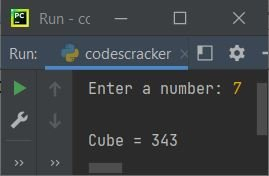Here is another sample run with user input "codescracker." This time, the input is actually a string, which cannot be converted into an int type value. Therefore, the output will be:Now the question is, what if the program is created without using try-except? Let's find out the answer using the practical program given below:

```print("Enter a number: ", end="")
num = input()

num = int(num)
cub = num*num*num
print("\nCube =", cub)```

Now the output with user input other than a number, say #, will be: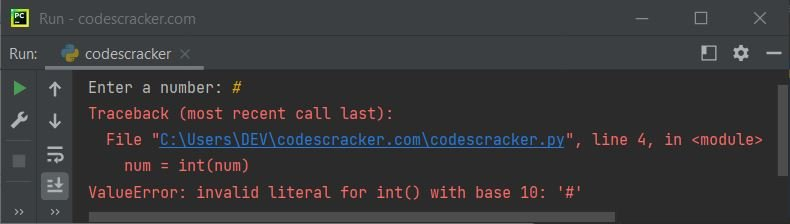As you can see from the above output, the program halts with the following statement:

`num = int(num)`

The name of the raised error or exception is ValueError. Therefore, we can catch this exception by using the try-except block in this way:

```print("Enter a number: ", end="")

try:
num = int(input())
print("\nCube =", num*num*num)
except ValueError:
print("\nInvalid Input!")```

I've also done a little modification to shorten the code. The above code will still produce the same output.

To produce only the default error message manually, create the program in this way:

```print("Enter a number: ", end="")

try:
num = int(input())
print("\nCube =", num*num*num)
except ValueError as ve:
print("\nInvalid Input!")
print("Exception:", ve)```

Now the output with user input # will be:### Multiple Exceptions with the Same Code in Python

We can use multiple except blocks to catch multiple exceptions raised by the code placed in the try block. For example:

```try:
print("The square of", num, "is", num*num)
except NameError:
print("The variable 'num' is not defined")
except:
print("Something went wrong!")```

since the variable num is not defined. Therefore, when we use an undefined variable/object in Python, it raises an exception named NameError. Therefore, the output will be:

`The variable 'num' is not defined`

Now let's modify the above program with the program given below:

```num = "10"
try:
num = int(num)
print("The square of", num, "is", num*num)
except NameError:
print("The variable 'num' is not defined")
except ValueError:
print("Invalid value stored in variable 'num'")
except:
print("Something went wrong!")```

since the variable num is defined before its use. Therefore, the output will be:

`The square of 10 is 100`

Let's again modify the above program. That is, initialize anything other than a number, say, Python to num. For example:

```num = "Python"
try:
num = int(num)
print("The square of", num, "is", num*num)
except NameError as ne:
print("The variable 'num' is not defined")
print("Exception:", ne)
except ValueError as ve:
print("Invalid value stored in variable 'num'")
print("Exception:", ve)
except:
print("Something went wrong!")```

Now the output should be:

```Invalid value stored in variable 'num'
Exception: invalid literal for int() with base 10: 'Python'```

These are just some demos showing the handling of multiple exceptions in Python.

#### The Syntax to Handle Multiple Exceptions

```try:
# do something
except exceptionName:
# handle particular exception
except (exceptionOne, exceptionTwo, exceptionThree):
# handle multiple exceptions at once
except:
# handle all other (remaining) exceptions```

## Python "try" with "finally"

The finally keyword is used to create a block of code to execute after the try...except block. This finally block of code will get executed, even if try raises an exception. For example:

```print("Enter a Number to Print its Table: ", end="")
try:
num = int(input())
print("\nTable of", num, ":")
for i in range(1, 11):
print(i*num)
except ValueError:
print("\nInvalid Input.")
finally:
print("\nProgram finished.")```

The sample run with user input 6 is: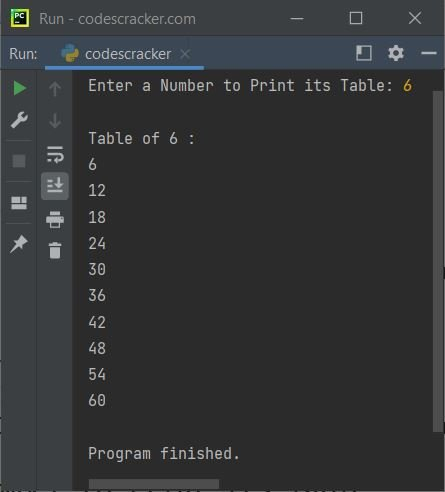And the screenshot below depicts another sample run with user input of \$.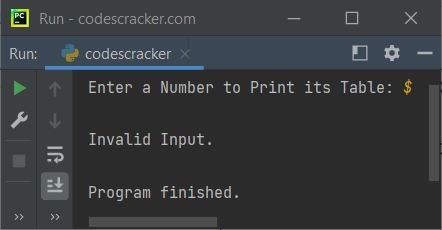Note: The finally block always executes, regardless of the result of try...except

## Python "try" with "else"

When no errors are raised, the else block following the try...except can be used to execute statement(s). For example:

```print("Enter a Number: ", end="")
try:
num = int(input())
except ValueError:
print("\nInvalid Input.")
else:
print("\nTable of", num, ":")
for i in range(1, 11):
print(num, "*", i, "=", num*i)```

The sample run with user input 8 is shown in the snapshot given below: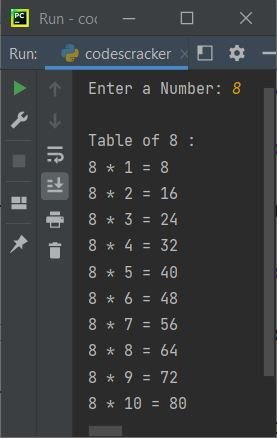whereas the sample run with invalid user input, say "fun," is shown in another snapshot, given below: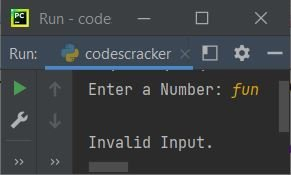## Python "raise" Keyword Example

The following program shows an example of the raise keyword in Python:

```print("Enter a Positive Number: ", end="")

try:
num = int(input())
if num<0:
raise Exception("Negative numbers aren't allowed.")
except ValueError:
print("\nInvalid Input!")```

The sample run with user input of -34, a negative number, is shown in the snapshot given below: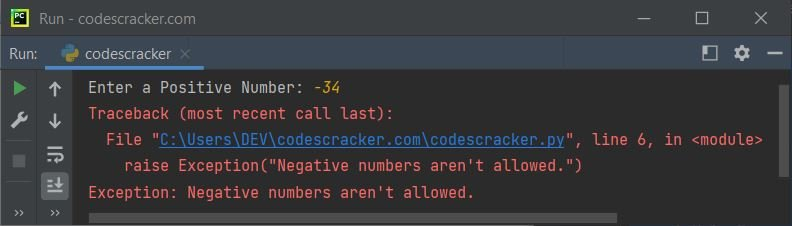Here is another example that demonstrates the raise statement or keyword in Python:

```print("Enter a Number: ", end="")
num = input()

if not type(num) is int:
raise TypeError("Invalid Input!")```

The sample run with user input "coding" is shown in the following snapshot: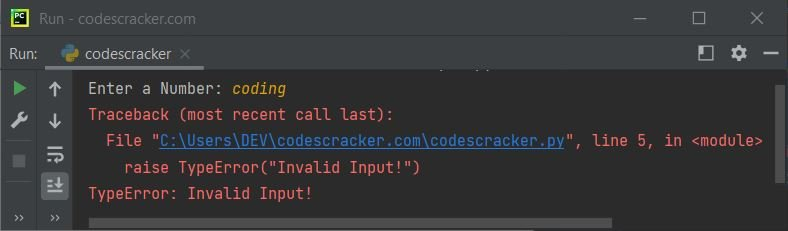Python Online Test

« Previous Tutorial Next Tutorial »